10.4 Images and Graphics

There's more to documents than text. Most PDF files contain some type of logo, diagram, illustration, or picture. This section shows how to include image files, build your own line-art illustrations, and repeat elements on every page (for instance, a header with a logo).

10.4.1 Images

PDF supports many different embedded image formats: PNG, JPEG, GIF, TIFF, CCITT, and a raw image format that consists of a stream of the exact byte sequence of pixels. Not every feature of every format is supported, however.

For PNG images, the alpha channel is lost (however, the later versions of pdflib and Acrobat do support transparency, which means that you can indicate a color index to be the transparent color, but you cannot have partial transparency). For JPEG, you only need to watch out for progressive JPEGs; they are not supported prior to Acrobat 4, so it is a good idea to stick to nonprogressive JPEGs. For GIF images, avoid interlacing.

Adding an image to a PDF document is relatively simple. The first step is to call the appropriate open function for the type of image you are using. These functions all take the form pdf_open_ format( ). For instance:

\$image = pdf_open_jpeg(pdf, filename);

Once you have opened the image, use pdf_place_image( ) to indicate where in your document the image should be located. While you have an image open, you can place it multiple times throughout your document; your generated file will contain only one copy of the actual image data. When you are done placing your image, call the pdf_close_image( ) function:

pdf_place_image(pdf, image, x, y, scale); pdf_close_image(pdf, image);

The scale parameter indicates the proportional scaling factor to be used when placing the image in the document.

You can get the dimensions of an image via pdf_get_value( ) calls on the imagewidth and imageheight keywords.

Example 10-7 places an image in several places on a page.

Example 10-7. Placing and scaling images
<?php  \$p = pdf_new(  );   pdf_open_file(\$p);   pdf_set_info(\$p,"Creator","images.php");   pdf_set_info(\$p,"Author","Rasmus Lerdorf");   pdf_set_info(\$p,"Title","Images");   pdf_begin_page(\$p,612,792);  \$im = pdf_open_jpeg(\$p, "php-big.jpg");  pdf_place_image(\$p, \$im, 200, 700, 1.0);  pdf_place_image(\$p, \$im, 200, 600, 0.75);  pdf_place_image(\$p, \$im, 200, 535, 0.50);  pdf_place_image(\$p, \$im, 200, 501, 0.25);  pdf_place_image(\$p, \$im, 200, 486, 0.10);  \$x = pdf_get_value(\$p, "imagewidth", \$im);  \$y = pdf_get_value(\$p, "imageheight", \$im);  pdf_close_image (\$p,\$im);  \$font = pdf_findfont(\$p,'Helvetica-Bold','host',0);  pdf_setfont(\$p,\$font,38.0);  pdf_show_xy(\$p,"\$x by \$y",425,750);  pdf_end_page(\$p);   pdf_close(\$p);   \$buf = pdf_get_buffer(\$p);   \$len = strlen(\$buf);  header("Content-Type: application/pdf");  header("Content-Length: \$len");   header("Content-Disposition: inline; filename=images.pdf");  echo \$buf;   pdf_delete(\$p);  ?>

Figure 10-7 shows the output of Example 10-7.

Figure 10-7. Placed and scaled imagesThe scaled versions of the PHP logo in Example 10-7 kept their original proportions. To do nonproportional scaling of an image, you must temporarily scale the coordinate system via a call to pdf_scale( ):

pdf_scale(pdf, xscale, yscale);

All subsequent coordinates will be multiplied by the xscale and yscale values.

Example 10-8 shows nonproportional scaling in action. Note that we had to compensate for the coordinate system scaling in the pdf_place_image( ) call to have the image show up in the right place.

Example 10-8. Nonproportional scaling
<?php   \$im = pdf_open_jpeg(\$p, "php-big.jpg");  pdf_place_image(\$p, \$im, 200, 700, 1.0);  pdf_save(\$p);  // Save current coordinate system settings  \$nx = 50/pdf_get_value(\$p,"imagewidth",\$im);  \$ny = 100/pdf_get_value(\$p,"imageheight",\$im);  pdf_scale(\$p, \$nx, \$ny);  pdf_place_image(\$p, \$im, 200/\$nx, 600/\$ny, 1.0);  pdf_restore(\$p);  // Restore previous  pdf_close_image (\$p,\$im); ?>

The output of Example 10-8 is shown in Figure 10-8.

Figure 10-8. Nonproportional scaling10.4.2 Graphics

To draw a graphical shape, first specify a path and then fill and/or stroke the path with appropriately configured fill and/or stroke colors. The functions that define these paths are straightforward. For example, to draw a line, you position the cursor at the starting point of the line using a call to pdf_moveto( ) , then specify the path for this line with a call to pdf_lineto( ). The starting points of other functions, such as pdf_circle( ) and pdf_rect( ), are defined directly in the calls.

The pdf_moveto( ) function starts the path at a particular point:

pdf_moveto(pdf, x, y);

With pdf_lineto( ), you can draw a line from the current point to another point:

pdf_lineto(pdf, x, y);

Use pdf_circle( ) to draw a circle of radius r at a particular point:

pdf_circle(pdf, x, y, r);

The pdf_arc( ) function draws an arc of a circle:

pdf_arc(pdf, x, y, r, alpha, beta);

The circle is centered at (x,y) and has radius r. The starting point of the arc is alpha degrees (measured counterclockwise from the horizontal axis), and the endpoint is beta degrees.

Use pdf_curveto( ) to draw a Bézier curve from the current point:

pdf_curveto(pdf, x1, y1, x2, y2, x3, y3);

The points (x1,y1), (x2,y2), and (x3,y3) are control points through which the curve must pass.

You can draw a rectangle with pdf_rect( ):

pdf_rect(pdf, x, y, width, height);

To draw a line from the current point back to the point that started the path, use pdf_closepath( ):

pdf_closepath(pdf);

Example 10-9 defines a simple path and strokes it.

Example 10-9. A simple graphic path
<?php  \$p = pdf_new(  );  pdf_open_file(\$p);  pdf_begin_page(\$p,612,792);  pdf_moveto(\$p,150,150);  pdf_lineto(\$p,450,650);  pdf_lineto(\$p,100,700);  pdf_curveto(\$p,80,400,70,450,250,550);  pdf_stroke(\$p);  pdf_end_page(\$p);  pdf_close(\$p);  \$buf = pdf_get_buffer(\$p);  \$len = strlen(\$buf);  header("Content-Type:application/pdf");  header("Content-Length:\$len");  header("Content-Disposition:inline; filename=gra.pdf");  echo \$buf;  pdf_delete(\$p); ?>

The output of Example 10-9 is shown in Figure 10-9.

Figure 10-9. A sample path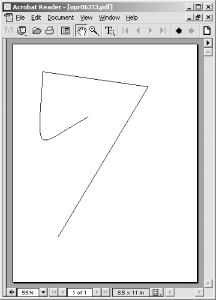We can use pdf_closepath( ) and pdf_fill_stroke( ) to close the path and then fill it with the current fill color by replacing the pdf_stroke( ) call in Example 10-9 with these two lines:

pdf_closepath(\$p); pdf_fill_stroke(\$p);

The pdf_fill_stroke( ) function fills and strokes the path with the current fill and stroke colors. Our output now looks like Figure 10-10.

Figure 10-10. Closed and filled path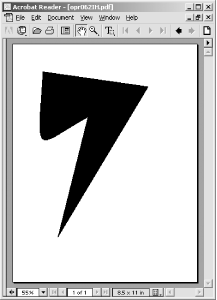Here's some code that experiments with different shapes and stroking or filling. Its output is shown in Figure 10-11.

// circle pdf_setcolor(\$p,"fill","rgb", 0.8, 0.5, 0.8); pdf_circle(\$p,400,600,75); pdf_fill_stroke(\$p); // funky arc pdf_setcolor(\$p,"fill","rgb", 0.8, 0.5, 0.5); pdf_moveto(\$p,200,600); pdf_arc(\$p,300,600,50,0,120); pdf_closepath(\$p); pdf_fill_stroke(\$p); // dashed rectangle pdf_setcolor(\$p,"stroke","rgb", 0.3, 0.8, 0.3); pdf_setdash(\$p,4,6); pdf_rect(\$p,50,500,500,300); pdf_stroke(\$p);
Figure 10-11. Different shapes and stroking and filling styles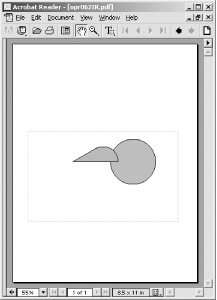10.4.3 Patterns

A pattern is a reusable component, defined outside of a page context, that is used in place of a color for filling or stroking a path.

The pdf_begin_pattern( ) call returns a pattern handle:

\$pattern = pdf_begin_pattern(pdf, width, height, xstep, ystep, painttype);

The width and height parameters specify the size of the pattern. If you are creating a pattern from an image, these are the dimensions of the image. The xstep and ystep parameters specify the horizontal and vertical tiling spacing (i.e., the distance between repetitions of the image). To tile the image without a gap between repetitions, set the xstep and ystep arguments to the same values as width and height. The final argument, painttype, can be either 1 or 2. 1 means that the pattern supplies its own color information. 2 means that the current fill and stroke colors are used instead. Patterns based on images only use a painttype of 1.

Example 10-10 creates a pattern from a small PHP logo image and uses it to fill a circle.

Example 10-10. Filling with a pattern
<?php  \$p = pdf_new(  );  pdf_open_file(\$p);  \$im = pdf_open_jpeg(\$p, "php-tiny.jpg");  \$pattern = pdf_begin_pattern(\$p,64,34,64,34,1);  pdf_save(\$p);  pdf_place_image(\$p, \$im, 0,0,1);  pdf_restore(\$p);  pdf_end_pattern(\$p);  pdf_close_image (\$p,\$im);  pdf_begin_page(\$p,612,792);  pdf_setcolor(\$p, "fill", "pattern", \$pattern);  pdf_setcolor(\$p, "stroke", "pattern", \$pattern);  pdf_setlinewidth(\$p, 30.0);  pdf_circle(\$p,306,396,120);  pdf_stroke(\$p);  pdf_end_page(\$p);  pdf_close(\$p);  \$buf = pdf_get_buffer(\$p);  \$len = strlen(\$buf);  Header("Content-Type:application/pdf");  Header("Content-Length: \$len");  Header("Content-Disposition: inline; filename=pat.pdf");  echo \$buf;  pdf_delete(\$p); ?>

The output of Example 10-10 is shown in Figure 10-12.

Figure 10-12. Pattern filling a circle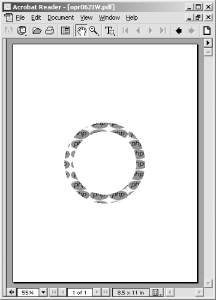10.4.4 Templates

It is common to have parts of a document, such as header/footer sections or background watermarks, repeated on multiple pages. It would be trivial to write a little PHP function to generate such things on each page, but if you did this the final PDF file would end up containing the same sequence of PDF calls on every page. PDF has built-in functionality known as "Form XObjects" (renamed "Templates" in pdflib) to more efficiently handle repeating elements.

To create a template, simply call pdf_begin_template( ), perform the various operations to create the PDF components you want this template to contain, then call pdf_end_template( ). It is a good idea to do a pdf_save( ) right after beginning the template and a pdf_restore( ) just before ending it to make sure that any context changes you perform in your template don't leak out of this template into the rest of the document.

The pdf_begin_template( ) function takes the dimensions of the template and returns a handle for the template:

\$template = pdf_begin_template(pdf, width, height);

The pdf_end_template( ), pdf_save( ), and pdf_restore( ) functions take no arguments beyond the pdf handle:

pdf_end_template(pdf); pdf_save(pdf); pdf_restore(pdf);

Example 10-11 uses templates to create a two-page document with the PHP logo in the top-left and top-right corners and the title "pdf Template Example" and a line at the top of each page. If you wanted to add something like a page number to your header, you would need to do that on each page. There is no way to put variable content in a template.

Example 10-11. Using a template
<?php  \$p = pdf_new(  );   pdf_open_file(\$p);   // define template  \$im = pdf_open_jpeg(\$p, "php-big.jpg");  \$template = pdf_begin_template(\$p,612,792);  pdf_save(\$p);  pdf_place_image(\$p, \$im, 14, 758, 0.25);  pdf_place_image(\$p, \$im, 562, 758, 0.25);  pdf_moveto(\$p,0,750);  pdf_lineto(\$p,612,750);  pdf_stroke(\$p);  \$font = pdf_findfont(\$p,"Times-Bold","host",0);  pdf_setfont(\$p,\$font,38.0);  pdf_show_xy(\$p,"pdf Template Example",120,757);  pdf_restore(\$p);  pdf_end_template(\$p);  pdf_close_image (\$p,\$im);// build pages  pdf_begin_page(\$p,595,842);   pdf_place_image(\$p, \$template, 0, 0, 1.0);  pdf_end_page(\$p);   pdf_begin_page(\$p,595,842);   pdf_place_image(\$p, \$template, 0, 0, 1.0);  pdf_end_page(\$p);   pdf_close(\$p);    \$buf = pdf_get_buffer(\$p);   \$len = strlen(\$buf);  header("Content-Type: application/pdf");  header("Content-Length: \$len");   header("Content-Disposition: inline; filename=templ.pdf");  echo \$buf;   pdf_delete(\$p);  ?>

The output of Example 10-11 is shown in Figure 10-13.

Figure 10-13. A templated page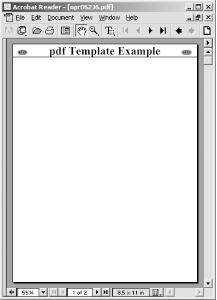Some operations, such as opening an image, cannot be done within the context of a template definition. Attempting to do so will cause an error. If you get such an error, simply move the offending operation to just before the pdf_begin_template( ) call.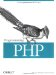Programming PHP
ISBN: 1565926102
EAN: 2147483647
Year: 2007
Pages: 168

Similar book on Amazon